Author: Oscar Cronquist Article last updated on January 03, 2023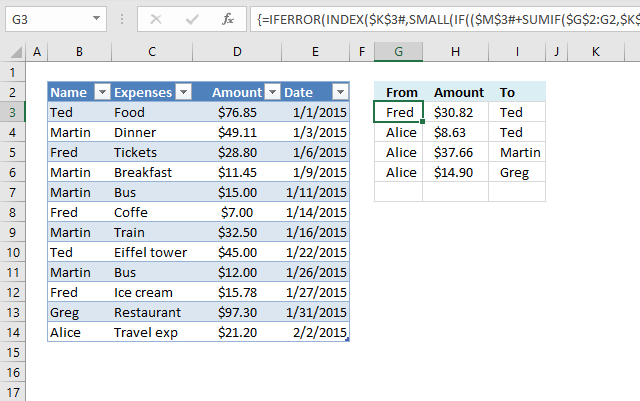This article demonstrates two ways to calculate expenses evenly split across multiple people. The first one is a formula solution, see image above and the second one is a VBA macro solution.

## 1. How to split expenses evenly (Excel 365)Everything is calculated automatically, the only thing you need to enter is the expenses each person has, see Excel Table in the image above. The date and Expenses columns are not really needed for the calculations.

The formulas in cell G3:H3, and I3 calculates how much each person needs to pay and to who to split expenses evenly based on the amounts in the Excel Table.

Excel 365 dynamic array formula in cell G3:

=IFERROR(INDEX(\$K\$3#, SMALL(IF((\$M\$3#+SUMIF(\$G\$2:G2, \$K\$3#, \$H\$2:H2))<0, SEQUENCE(COUNT(\$M\$3#)), ""), 1)), "")

Excel 365 dynamic array formula in cell H3:

=LET(w,\$H\$2:H2,z,\$K\$3#,x,SUMIF(\$G\$2:G2,z,w),y,SUMIF(\$I\$2:I2,z,w),IFERROR(MIN(ABS(FILTER(\$M\$3#+x-y,z=G3)),ABS(FILTER(\$M\$3#+x-y,z=I3))),""))

Excel 365 dynamic array formula in cell I3:

=IFERROR(INDEX(\$K\$3#, SMALL(IF((\$M\$3#-SUMIF(\$I\$2:I2, \$K\$3#, \$H\$2:H2))>0.1, SEQUENCE(COUNT(\$M\$3#)), ""), 1)), "")

The formulas above contain Excel 365 functions and work only in Excel 365, they use values from columns K to M, see image below.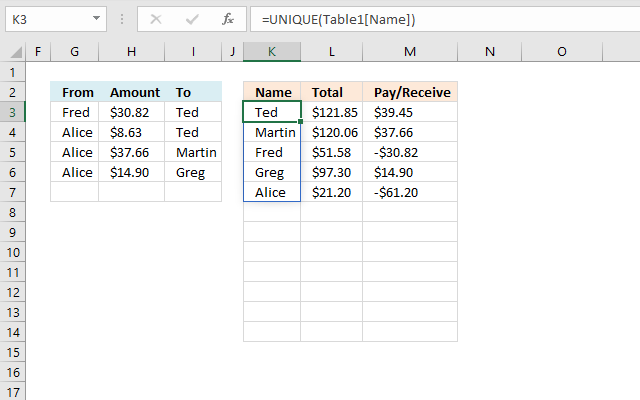Excel 365 dynamic array formula in cell K3:

=UNIQUE(Table1[Name])

Formula in cell L3:

=SUMIF(Table1[Name],K3#,Table1[Amount])

Excel 365 dynamic array formula in cell M3:

=L3#-SUM(Table1[Amount])/COUNTA(UNIQUE(Table1[Name]))

### Explaining the formula in cell G3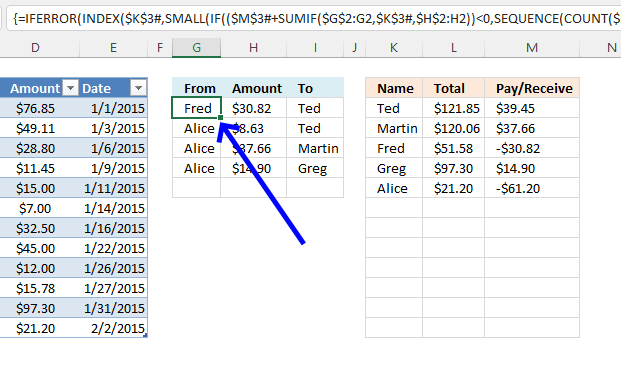This formula calculates which names will have to pay.

#### Step 1 - Sum amounts based on conditions

The SUMIF function sums numerical values based on a condition.

Function syntax: SUMIF(range, criteria, [sum_range])

SUMIF(\$G\$2:G2, \$K\$3#, \$H\$2:H2)

becomes

SUMIF("From",{"Ted";"Martin";"Fred";"Greg";"Alice"},"Amount")

and returns

{0; 0; 0; 0; 0}.

#### Step 2 - Add values

The plus sign lets you add numbers in an Excel formula.

\$M\$3#+SUMIF(\$G\$2:G2, \$K\$3#, \$H\$2:H2)

becomes

{39.453; 37.663; -30.822; 14.903; -61.197} + {0; 0; 0; 0; 0}

and returns

{39.453; 37.663; -30.822; 14.903; -61.197}

#### Step 3 - Check if sums are lower than 0 (zero)

The less than sign lets you check if a number is smaller than another number, the reuslt is a boolean value TRUE or FALSE.

(\$M\$3#+SUMIF(\$G\$2:G2, \$K\$3#, \$H\$2:H2))<0

becomes

{39.453; 37.663; -30.822; 14.903; -61.197}<0

and returns

{FALSE;FALSE;TRUE;FALSE;TRUE}.

#### Step 4 - Count numbers in the spilled formula

The COUNT function counts all numerical values in an argument.

Function syntax: COUNT(value1, [value2], ...)

COUNT(\$M\$3#)

becomes

COUNT({39.453;37.663;-30.822;14.903;-61.197})

and returns

5.

#### Step 5 - Create a sequence from 1 to n

The SEQUENCE function creates a list of sequential numbers.

Function syntax: SEQUENCE(rows, [columns], [start], [step])

SEQUENCE(COUNT(\$M\$3#))

becomes

SEQUENCE(5)

and returns

{1; 2; 3; 4; 5}.

#### Step 6 - Replace numbers below zero with the corresponding number in the sequence array

The IF function returns one value if the logical test is TRUE and another value if the logical test is FALSE.

Function syntax: IF(logical_test, [value_if_true], [value_if_false])

IF((\$M\$3#+SUMIF(\$G\$2:G2, \$K\$3#, \$H\$2:H2))<0, SEQUENCE(COUNT(\$M\$3#)), "")

becomes

IF({FALSE;FALSE;TRUE;FALSE;TRUE}, {1; 2; 3; 4; 5}, "")

and returns

{""; ""; 3; ""; 5}.

#### Step 7 - Extract the smallest number in the array

The SMALL function returns the k-th smallest value from a group of numbers.

Function syntax: SMALL(array, k)

SMALL(IF((\$M\$3#+SUMIF(\$G\$2:G2, \$K\$3#, \$H\$2:H2))<0, SEQUENCE(COUNT(\$M\$3#)), ""), 1)

becomes

SMALL({""; ""; 3; ""; 5}, 1)

and returns

3.

#### Step 8 - Get value from the spilled formula in cell \$K\$3#

The INDEX function returns a value or reference from a cell range or array, you specify which value based on a row and column number.

Function syntax: INDEX(array, [row_num], [column_num])

INDEX(\$K\$3#, SMALL(IF((\$M\$3#+SUMIF(\$G\$2:G2, \$K\$3#, \$H\$2:H2))<0, SEQUENCE(COUNT(\$M\$3#)), ""), 1))

becomes

INDEX(\$K\$3#, 3)

becomes

INDEX({"Ted";"Martin";"Fred";"Greg";"Alice"}, 3)

and returns

"Fred".

#### Step 9 - Remove possible errors

The IFERROR function if the value argument returns an error, the value_if_error argument is used. If the value argument does NOT return an error, the IFERROR function returns the value argument.

Function syntax: IFERROR(value, value_if_error)

IFERROR(INDEX(\$K\$3#, SMALL(IF((\$M\$3#+SUMIF(\$G\$2:G2, \$K\$3#, \$H\$2:H2))<0, SEQUENCE(COUNT(\$M\$3#)), ""), 1)), "")

becomes

IFERROR("Fred", "")

and returns

"Fred".

### Explaining the formula in cell H3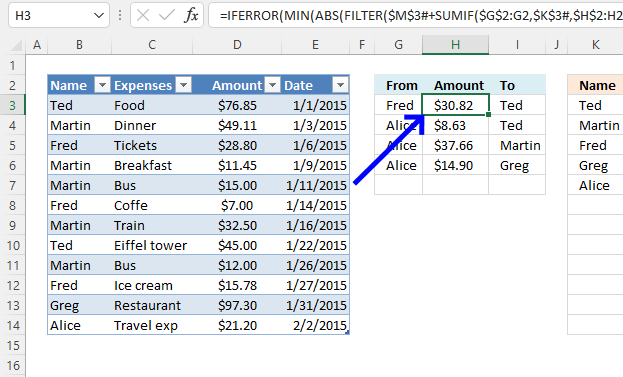This formula calculates the amount to pay.

#### Step 1 - Sum based on a condition

The SUMIF function sums numerical values based on a condition.

Function syntax: SUMIF(range, criteria, [sum_range])

\$G\$2:G2 and \$H\$2:H2 are cell references containing both a relative and absolute part indicated by the \$ dollar signs. This makes them grow when the formula cell is copied to the cells below.

\$K\$3# is a cell reference to cell K3, however, the # (hashtag) makes it reference all spilled values in the Excel 365 dynamic array formula located in cell K3.

SUMIF(\$G\$2:G2,\$K\$3#,\$H\$2:H2)

becomes

SUMIF("From",{"Ted";"Martin";"Fred";"Greg";"Alice"},"Amount")

and returns

{0;0;0;0;0}.

#### Step 2 - Sum based on a condition

The SUMIF function sums numerical values based on a condition.

Function syntax: SUMIF(range, criteria, [sum_range])

SUMIF(\$I\$2:I2,\$K\$3#,\$H\$2:H2)

becomes

SUMIF("To",{"Ted";"Martin";"Fred";"Greg";"Alice"},"Amount")

and returns

{0;0;0;0;0}.

#### Step 3 - Subtract arrays

The minus character lets you subtract numbers in an Excel formula.

SUMIF(\$G\$2:G2,\$K\$3#,\$H\$2:H2)-SUMIF(\$I\$2:I2,\$K\$3#,\$H\$2:H2)

becomes

{0;0;0;0;0} - {0;0;0;0;0}

and returns

{0;0;0;0;0}

#### Step 4 - Compare

The equal sign lets you compare values in an Excel formula. The result is a boolean value TRUE or FALSE.

\$K\$3#=G3

becomes

{"Ted";"Martin";"Fred";"Greg";"Alice"}="Fred"

and returns

{FALSE;FALSE;TRUE;FALSE;FALSE}.

#### Step 5 - Filter values

The FILTER function extracts values/rows based on a condition or criteria.

Function syntax: FILTER(array, include, [if_empty])

FILTER(\$M\$3#+SUMIF(\$G\$2:G2,\$K\$3#,\$H\$2:H2)-SUMIF(\$I\$2:I2,\$K\$3#,\$H\$2:H2),\$K\$3#=G3)

becomes

FILTER({39.453;37.663;-30.822;14.903;-61.197}+{0;0;0;0;0}-{0;0;0;0;0},{FALSE;FALSE;TRUE;FALSE;FALSE})

becomes

FILTER({39.453;37.663;-30.822;14.903;-61.197},{FALSE;FALSE;TRUE;FALSE;FALSE})

and returns

-30.822

#### Step 6 - Filter values

The FILTER function extracts values/rows based on a condition or criteria.

Function syntax: FILTER(array, include, [if_empty])

FILTER(\$M\$3#+SUMIF(\$G\$2:G2,\$K\$3#,\$H\$2:H2)-SUMIF(\$I\$2:I2,\$K\$3#,\$H\$2:H2),\$K\$3#=I3)

becomes

FILTER({39.453;37.663;-30.822;14.903;-61.197}+{0;0;0;0;0}-{0;0;0;0;0},\$K\$3#=I3)

becomes

FILTER({39.453;37.663;-30.822;14.903;-61.197},{"Ted";"Martin";"Fred";"Greg";"Alice"}="Ted")

becomes

FILTER({39.453;37.663;-30.822;14.903;-61.197},{TRUE;FALSE;FALSE;FALSE;FALSE})

and returns

39.453

#### Step 7 - Remove the negative sign

The ABS function converts negative numbers to positive numbers.

Function syntax: ABS(number)

ABS(FILTER(\$M\$3#+SUMIF(\$G\$2:G2,\$K\$3#,\$H\$2:H2)-SUMIF(\$I\$2:I2,\$K\$3#,\$H\$2:H2),\$K\$3#=G3))

becomes

ABS(-30.822)

and returns

30.822

#### Step 8 - Remove the negative sign

The ABS function converts negative numbers to positive numbers.

Function syntax: ABS(number)

ABS(FILTER(\$M\$3#+SUMIF(\$G\$2:G2,\$K\$3#,\$H\$2:H2)-SUMIF(\$I\$2:I2,\$K\$3#,\$H\$2:H2),\$K\$3#=I3))

becomes

ABS(39.453)

and returns

39.453

#### Step 9 - Get the smallest number

The MIN function returns the smallest number in a cell range.

Function syntax: MIN(number1, [number2], ...)

MIN(ABS(FILTER(\$M\$3#+SUMIF(\$G\$2:G2,\$K\$3#,\$H\$2:H2)-SUMIF(\$I\$2:I2,\$K\$3#,\$H\$2:H2),\$K\$3#=G3)),ABS(FILTER(\$M\$3#+SUMIF(\$G\$2:G2,\$K\$3#,\$H\$2:H2)-SUMIF(\$I\$2:I2,\$K\$3#,\$H\$2:H2),\$K\$3#=I3)))

becomes

MIN(30.822, 39.453)

and returns

30.822

#### Step 10 - Remove error values

The IFERROR function if the value argument returns an error, the value_if_error argument is used. If the value argument does NOT return an error, the IFERROR function returns the value argument.

Function syntax: IFERROR(value, value_if_error)

IFERROR(MIN(ABS(FILTER(\$M\$3#+SUMIF(\$G\$2:G2,\$K\$3#,\$H\$2:H2)-SUMIF(\$I\$2:I2,\$K\$3#,\$H\$2:H2),\$K\$3#=G3)),ABS(FILTER(\$M\$3#+SUMIF(\$G\$2:G2,\$K\$3#,\$H\$2:H2)-SUMIF(\$I\$2:I2,\$K\$3#,\$H\$2:H2),\$K\$3#=I3))),"")

becomes

IFERROR(30.822,"")

and returns

30.822

#### Step 11 - Shorten the formula

The LET function lets you name intermediate calculation results which can shorten formulas considerably and improve performance.

Function syntax: LET(name1, name_value1, calculation_or_name2, [name_value2, calculation_or_name3...])

IFERROR(MIN(ABS(FILTER(\$M\$3#+SUMIF(\$G\$2:G2,\$K\$3#,\$H\$2:H2)-SUMIF(\$I\$2:I2,\$K\$3#,\$H\$2:H2),\$K\$3#=G3)),ABS(FILTER(\$M\$3#+SUMIF(\$G\$2:G2,\$K\$3#,\$H\$2:H2)-SUMIF(\$I\$2:I2,\$K\$3#,\$H\$2:H2),\$K\$3#=I3))),"")

w - \$H\$2:H2,z,\$K\$3#

x - SUMIF(\$G\$2:G2,z,w)

y - SUMIF(\$I\$2:I2,z,w)

LET(w,\$H\$2:H2,z,\$K\$3#,x,SUMIF(\$G\$2:G2,z,w),y,SUMIF(\$I\$2:I2,z,w),IFERROR(MIN(ABS(FILTER(\$M\$3#+x-y,z=G3)),ABS(FILTER(\$M\$3#+x-y,z=I3))),""))

### Explaining the formula in cell I3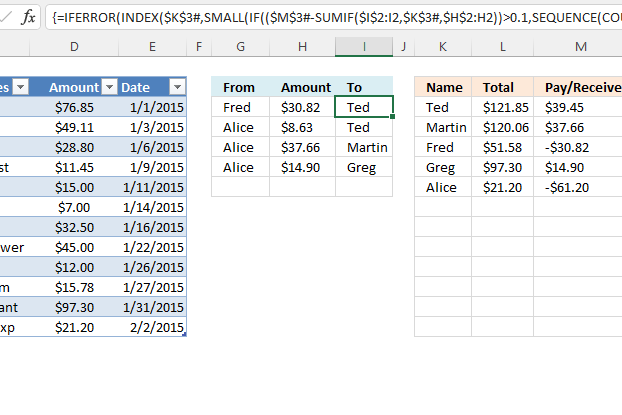This formula calculates who will receive the amount. The calculation is almost identical to the one in cell G3 except for steps 1 and 8.

#### Step 1 - Sum amounts based on conditions

The SUMIF function sums numerical values based on a condition.

Function syntax: SUMIF(range, criteria, [sum_range])

SUMIF(\$I\$2:I2, \$K\$3#, \$H\$2:H2)

becomes

SUMIF("To",{"Ted";"Martin";"Fred";"Greg";"Alice"},"Amount")

and returns

{0; 0; 0; 0; 0}.

#### Step 8 - Get value from the spilled formula in cell \$K\$3#

The INDEX function returns a value or reference from a cell range or array, you specify which value based on a row and column number.

Function syntax: INDEX(array, [row_num], [column_num])

INDEX(\$K\$3#, SMALL(IF((\$M\$3#+SUMIF(\$G\$2:G2, \$K\$3#, \$H\$2:H2))<0, SEQUENCE(COUNT(\$M\$3#)), ""), 1))

becomes

INDEX(\$K\$3#, 3)

becomes

INDEX({"Ted";"Martin";"Fred";"Greg";"Alice"}, 3)

and returns

"Fred".

### Explaining the formula in cell K3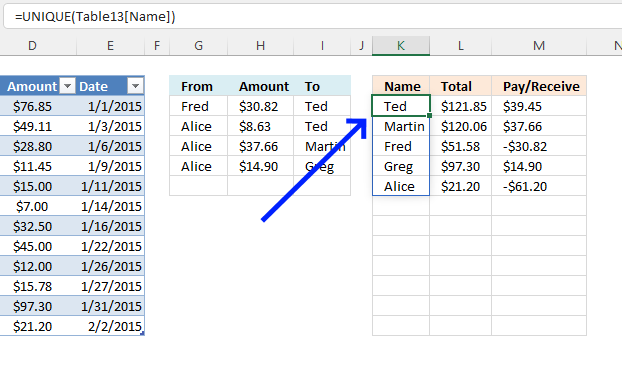This formula returns a list of unique distinct names, meaning no repeating names.

#### Step 1 - Create a structured reference to column Name in Table13

The easiest way to create this cell reference is to double-press with left mouse button on cell K3, type =, then select column Name in Excel Table Table13. Excel now creates the cell reference automatically for you.

Why use an Excel Table? The cell reference doesn't change when you add or remove values, you don't have to adjust the cell reference at all like regular cell references. A cell reference to an Excel table is called a structured reference.

Table13[Name]

#### Step 2 - List unique distinct values

The UNIQUE function returns a unique or unique distinct list.

Function syntax: UNIQUE(array,[by_col],[exactly_once])

UNIQUE(Table13[Name])

becomes

UNIQUE({"Ted"; "Martin"; "Fred"; "Martin"; "Martin"; "Fred"; "Martin"; "Ted"; "Martin"; "Fred"; "Greg"; "Alice"})

and returns

{"Ted"; "Martin"; "Fred"; "Greg"; "Alice"}.

### Explaining the formula in cell L3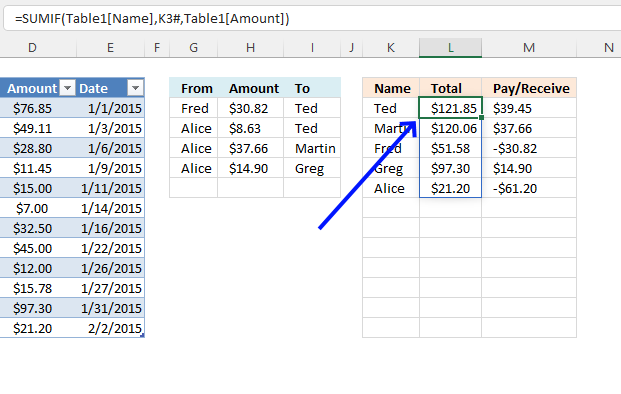This formula calculates the total each person has spent.

#### Step 1 - Populate arguments

The SUMIF function sums numerical values based on a condition.

Function syntax: SUMIF(range, criteria, [sum_range])

range - Table13[Name]

criteria - K3#

[sum_range] - Table13[Amount]

#### Step 2 - Evaluate SUMIF function

SUMIF(Table13[Name], K3#,Table13[Amount])

becomes

SUMIF({"Ted"; "Martin"; "Fred"; "Martin"; "Martin"; "Fred"; "Martin"; "Ted"; "Martin"; "Fred"; "Greg"; "Alice"},{"Ted"; "Martin"; "Fred"; "Greg"; "Alice"}, {76.85; 49.11; 28.8; 11.45; 15; 7; 32.5; 45; 12; 15.775; 97.3; 21.2})

and returns

{121.85; 120.06; 51.575; 97.3; 21.2}

### Explaining the formula in cell M3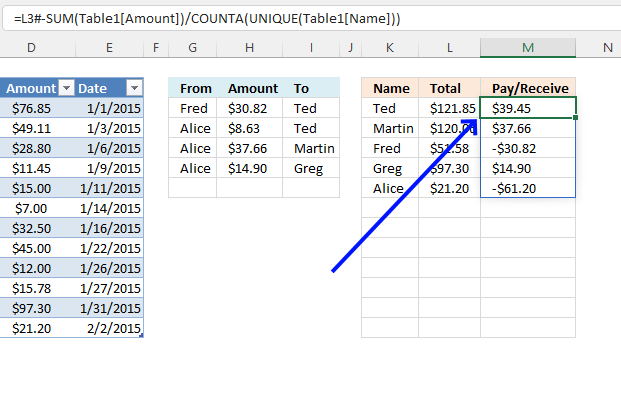This formula calculates the amount to be paid or received, a negative value meaning pay and a positive value meaning receive.

#### Step 1 - List unique distinct values

The UNIQUE function returns a unique or unique distinct list.

Function syntax: UNIQUE(array,[by_col],[exactly_once])

UNIQUE(Table13[Name])

becomes

UNIQUE({"Ted"; "Martin"; "Fred"; "Martin"; "Martin"; "Fred"; "Martin"; "Ted"; "Martin"; "Fred"; "Greg"; "Alice"})

and returns

{"Ted"; "Martin"; "Fred"; "Greg"; "Alice"}.

#### Step 2 - Count non-empty values

The COUNTA function counts the non-empty or non-blank cells in a cell range.

Function syntax: COUNTA(value1, [value2], ...)

COUNTA(UNIQUE(Table13[Name])

becomes

COUNTA({"Ted"; "Martin"; "Fred"; "Greg"; "Alice"})

and returns

5.

#### Step 3 - Add numbers and return a total

The SUM function allows you to add numerical values, the function returns the sum in the cell it is entered in. The SUM function is cleverly designed to ignore text and boolean values, adding only numbers.

Function syntax: SUM(number1, [number2], ...)

SUM(Table13[Amount])

becomes

SUM({76.85; 49.11; 28.8; 11.45; 15; 7; 32.5; 45; 12; 15.775; 97.3; 21.2})

and returns

411.985

#### Step 4 - Divide the sum with unique distinct count

The division sign lets you perform a division in an Excel formula.

SUM(Table13[Amount])/COUNTA(UNIQUE(Table13[Name]))

becomes

411.985/5

and returns

82.397

#### Step 5 - Subtract totals in column L with the quotient

The minus sign calculates a difference between two numbers in an Excel formula.

L3#-SUM(Table13[Amount])/COUNTA(UNIQUE(Table13[Name]))

becomes

{121.85;120.06;51.575;97.3;21.2} - 82.397

and returns

{39.453; 37.663; -30.822; 14.903; -61.197}.

### Get the Excel fileSplit-expensesv3.xlsx

## 2. How to split expenses evenly (VBA  Macro)This workbook lets you split expenses evenly with other people. Type name, expense, and amount in the Excel table on sheet 'Expenses'.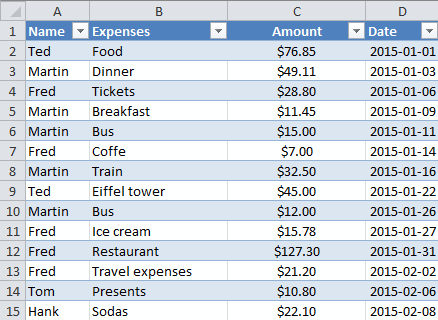Excel returns amounts to be paid and individuals involved. This is not the macro you are looking for if you want to calculate the smallest number of transactions possible.### How calculation sheet works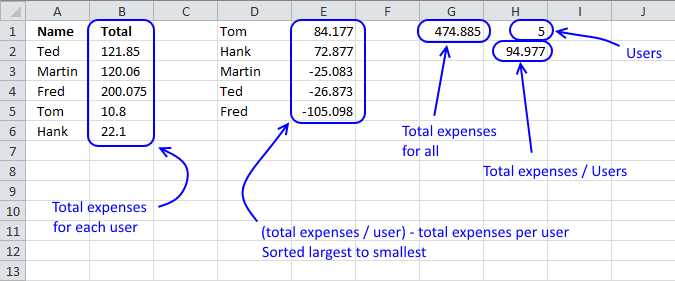The vba macro uses the values in cell range D1:E5 and calculates transactions so all sums even out.

For example, Tom pays Fred \$84.177 and his sum is 84.177 - 84.177 = 0. Hank pays Fred \$20.92, Ted \$26.87, Martin \$25.08 and his sum is 72.88 - 20.92 - 26.87 - 25.08 = 0.

Those were the necessary transactions to even out all user sums to zero. The remaining sums are now all 0, Martin's sum is -25.08 + 25.08 = 0, Ted -26.87 + 26.87 = 0 and Fred -105.098 + 84.177 + 20.92 = 0.

### How I made this workbook

There are two sheets in this workbook, 'Expenses' and 'Calculation'. Here are the formulas on 'Calculation' sheet.

Unique distinct names in column A:

=IFERROR(INDEX(Table1[Name],MATCH(0,COUNTIF(\$A\$1:A1,Table1[Name]),0)),"")

Read this post: How to extract a unique distinct list from a column

Sum values for each unique name in column B:

=IF(A2<>"",SUMIF(Table1[Name],A2,Table1[Amount]),"")

Count unique names in cell H1:

=MAX(IF(A2:A38<>"",MATCH(ROW(A2:A38),ROW(A2:A38)),""))

Sum amounts in cell G1:

=SUM(B2:B34)

VBA macro

```'Name macro
Sub SplitExp()

'Dimension variables and declare data types
Dim r As Single, d As Single, e As Single, Lrow As Single

'Disable screen refresh
Application.ScreenUpdating = False

'With ... End With statement
With Worksheets("Calculation")

'Save value in cell H1 to variable r
r = .Range("H1")

'Clear everything in columns D to E
.Columns("D:E").Clear

'Clear cell range F2:H100 in worksheet Expenses
Worksheets("Expenses").Range("F2:H100").Clear

'Save values from cell range A2:Br+1 to cell range D1:Er based on variable r
.Range("D" & 1 & ":E" & r) = .Range("A" & 2 & ":B" & r + 1).Value

'For ... Next statement
For d = 1 To r
.Range("E" & d) = (.Range("G1") / r) - .Range("E" & d)
Next d
For d = 1 To r
For e = r To 1 Step -1
Lrow = Worksheets("Expenses").Range("F" & Rows.Count).End(xlUp).Row + 1
If Round(.Range("E" & d), 2) <> 0 And Round(.Range("E" & e), 2) <> 0 Then
If Application.Min(Abs(.Range("E" & d)), Abs(.Range("E" & e))) = Abs(.Range("E" & d)) Then
Worksheets("Expenses").Range("F" & Lrow & ":H" & Lrow) = Array(.Range("D" & d), Round(Abs(.Range("E" & d)), 2), .Range("D" & e))
.Range("E" & e) = .Range("E" & e) + .Range("E" & d)
.Range("E" & d) = 0
Else
Worksheets("Expenses").Range("F" & Lrow & ":H" & Lrow) = Array(.Range("D" & d), Round(Abs(.Range("E" & e)), 2), .Range("D" & e))
.Range("E" & d) = .Range("E" & e) + .Range("E" & d)
.Range("E" & e) = 0
End If
End If
Next e
Next d
End With
Application.ScreenUpdating = True
End Sub

```

Event code, sheet 'Calculation'

```Private Sub Worksheet_Calculate()

Call SplitExp

End Sub
```